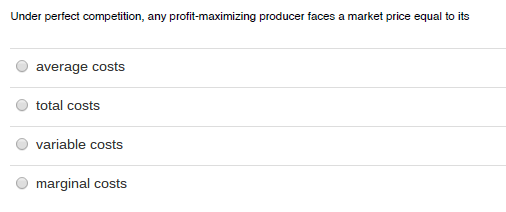# Under perfect competition. any profit-maximizing producer faces a market price equal to its

Under perfect competition, any profit-maximizing producer faces
a market equal to itsUnder perfect competition, any profit-maximizing producer faces a market price equal to its average costs total costs variable costs marginal costs

Hi,
The correct answer is Marginal Cost
Explanation: In perfect competition, any profit-maximizing
producer faces a market price equal to its marginal cost (P = MC).
This implies that a factor’s price equals the factor’s marginal
revenue product. It allows for derivation of the supply curve on
which the neoclassical approach is based.